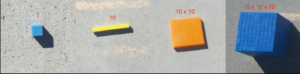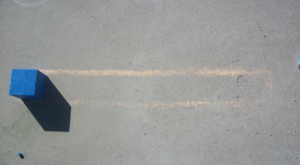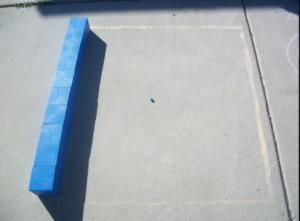We are all aware of the CRA model of providing students experiences with mathematics content. In terms of exponents, students very rarely experience the “C” (concrete) or the “R” (representational) and usually begin with the “A” (abstract). So how can we provide “C” and “R” experience?

Last week we shared a PowerPoint from CPalms with images for students to look at powers of ten. This blog post shares pictures of students using manipulatives to model the exponential value.Students should be familiar with base ten blocks. Which of these blocks could be used to represent 102? Let’s start by thinking which represents 10? (The ten rod/long) If the exponent 2 or “squared” means to multiply 10 x 10, how can we represent that? That’s right, a hundred flat. When you look down upon a flat, you even see the shape of a square!

Let’s move to 103. What multiplication problem are we talking about here? (10 x 10 x 10) How can we show that with blocks? We know a flat represents 10 x 10, so we need 10 flats. That’s one of the large cubes! We can begin to see a pattern:What exponents could we use to label our base ten blocks? What exponent works for the ones cube?
How can we represent 104? Each time we move to the next exponent of ten, we are basically creating another group of 10 of that model. So what would ten groups of large cubes look like? How about ten groups of that object for 105? This is a great challenge for students to represent both physically and through drawing.
Below are images one of our math coaches have captured from student work in this area:Here are some additional questions you can use with your students: What patterns do you see? What does the picture from the beginning of the blog post represent? How many ones cubes fit in each of these models? So what is the value of each of these new “super” base-ten blocks?